## 江苏卫生健康委公布最新传染病疫情

[处方门槛形同虚设] [江苏8月空气质量排名出炉]

## 老人被药房欺诈高价买药还遭恐吓

[男童吃蘑菇中毒致肝衰竭] [三位南京医生高铁上急救乘客]

## 微健康

• 名医@我
• 网友提问

2014-01-20 13:40:48

## 健康常识

• 今日排行
• 点击排行

"; windowOpener1(txt); } function count_color(c_year, c_month, j) { var c_tday = new Date(Date.UTC(c_year, c_month - 1, j)); c_sec = c_tday.getTime(); var temp_sec = l_sec; if (l_sec < c_sec) { while (temp_sec < c_sec) { temp_sec = temp_sec + t * msPerDay } temp_sec = temp_sec - t * msPerDay; var temp_time = new Date(); temp_time.setTime(temp_sec); var day_case = (c_sec - temp_time) / msPerDay; if (day_case >= 0 && day_case <= 4) { return "FF3300" } if (day_case >= 5 && day_case <= (t / 2 - 6)) { return "009933" } if (day_case >= (t / 2 - 5) && day_case <= (t / 2 + 4)) { return "FFC934" } if (day_case >= (t / 2 + 5) && day_case <= (t - 1)) { return "009933" } if (day_case == t) { return "FF3300" } } else if (c_sec == l_sec) { return "FF3300" } else if (c_sec < l_sec) { while (temp_sec > c_sec) { temp_sec = temp_sec - t * msPerDay } var temp_time = new Date(); temp_time.setTime(temp_sec); var day_case = (c_sec - temp_time) / msPerDay; if (day_case >= 0 && day_case <= 4) { return "FF3300" } if (day_case >= 5 && day_case <= (t / 2 - 6)) { return "009933" } if (day_case >= (t / 2 - 5) && day_case <= (t / 2 + 4)) { return "FFC934" } if (day_case >= (t / 2 + 5) && day_case <= (t - 1)) { return "009933" } if (day_case == t) { return "FF3300" } } } function out_calendar() { var firstday = new Date(Date.UTC(syear, smonth - 1, 1)); f_sec = firstday.getTime(); f_day = firstday.getDay(); txt = ""; txt = txt + ""; txt = txt + "
"; txt = txt + ""; txt = txt + ""; if (smonth == 12) { var nmonth = 1 var nyear = parseInt(syear) + 1; } else { var nyear = syear; var nmonth = parseInt(smonth) + 1; } var temp_sec = l_sec; if (f_sec > l_sec) { while (temp_sec < f_sec) { temp_sec = temp_sec + t * msPerDay; } temp_sec = temp_sec - t * msPerDay; var temp_time = new Date(); temp_time.setTime(temp_sec); var day_case = (f_sec - temp_time) / msPerDay; var day_case = s_mdays - t - day_case; } else if (s_sec == l_sec) { var day_case = s_mdays - t - lday; } else if (f_sec < l_sec) { while (temp_sec > s_sec) { temp_sec = temp_sec - t * msPerDay; } var temp_time = new Date(); temp_time.setTime(temp_sec); var day_case = (f_sec - temp_time) / msPerDay; var day_case = s_mdays - t - day_case; } if (day_case < 0) { firstday = new Date(Date.UTC(nyear, nmonth - 1, 1)); f_day = firstday.getDay(); s_mdays = month_days(nyear, nmonth); txt = txt + ""; } txt = txt + ""; txt = txt + "
" txt = txt + ""; txt = txt + ""; txt = txt + ""; line = 0; j = 1 s_mdays = month_days(syear, smonth); while (j <= s_mdays) { txt = txt + ""; for (i = 0; i <= 6; i++) { if (line == 0 && i < f_day) { txt = txt + ""; } else { txt_color = count_color(syear, smonth, j); txt = txt + ""; j++; } if (j > s_mdays) { break; } if (i == 6) { line = 1; } } txt = txt + ""; } txt = txt + "
 " + syear + "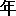" + smonth + "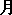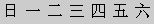" + j + "
"; txt = txt + ""; txt = txt + ""; txt = txt + ""; line = 0; j = 1 while (j <= s_mdays) { txt = txt + ""; for (i = 0; i <= 6; i++) { if (line == 0 && i < f_day) { txt = txt + ""; } else { txt_color = count_color(nyear, nmonth, j); txt = txt + ""; j++; } if (j > s_mdays) { break; } if (i == 6) { line = 1; } } txt = txt + ""; } txt = txt + "
 " + nyear + "" + nmonth + "" + j + "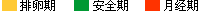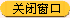"; txt = txt + ""; windowOpener1(txt); } function check_form(obj) { initWm(); if (isNaN(obj.value)) { alert("周期无效，请输入数字"); obj.focus() } else if (lday > month_days(lyear, lmonth)) { alert("所选日期无效，请重新输入") } else if (sday > month_days(syear, smonth)) { alert("所选日期无效，请重新输入") } else { // 080908 为监测流量所加 begin //urlStr = strClock(); //document.getElementById('weightPV').src = "http://eladies.sina.com.cn/misc/2008/0908/2101.html?" + urlStr; // 080908 为监测流量所加 end if (document.getElementById("aqq_datepicker_1").value==""||document.getElementById("aqq_datepicker_1").value=="点击选择日期") { alert("请选择上次月经时间") }else if (document.getElementById("aqq_datepicker_2").value==""||document.getElementById("aqq_datepicker_2").value=="点击选择日期") { alert("请选择查询时间") } else { out_case() } } }